# Methods of Speed Control of DC Motor

Posted on

How can the speed of a DC motor be controlled? One of the most useful features of DC motor control is the speed control. If you control the speeds of the motor, you need to vary the speed of the motor according to the requirements for getting the required operation. There are many applications like the movement of the robotic vehicles, motors in paper mills, and elevators use the speed control mechanism.

The speed of the DC motor can be controlled by some following method:

• By using rotor resistance starter
• By using 3 point starter
• By using 4 point starter
• By using field divider resistor for DC series motor
• By using armature divider resistor for Dc series motor
• By using variable supply voltage using chopper circuit
• Connecting series rheostat with field winding for Dc shunt motor
• Connecting series rheostat with armature winding for DC shunt motor.

These are the various method of controlling speed for DC motor.

# Methods of Speed Control of DC Motor

Speed control of DC motor is by two main methods.

1. Armature voltage control.
2. Field voltage control.

## Armature Voltage Control

There are two main methods for this.

1. Full control bridge.
2. Chopper control.

Full control bridge: This consist of six SCRs for three phase. Back to back full control bridge is used for four quadrant operation of the DC motor. i.e. forward/reverse driving with regenerative braking.Chopper Control: This is used for speed control of the battery operated vehicle. Pulse width modulation technique is used to control DC voltage given to DC motor.## Field Voltage Control

Field voltage control is used to raise separately excited DC motor speed above rated speed by keeping armature voltage constant. This is also called field weakening. Half control bridge is used for field voltage control as shown in above figure.

## What are the Best Ways to Control the Speed of DC Motor?

Speed control means intentional change of the drive speed to a value required for performing the specific work process. Speed control is a different concept from speed regulation where there is natural change in speed due change in load on the shaft. Speed control is either done manually by the operator or by means of some automatic control device.

One of the important features of DC motor is that its speed can be controlled with relative ease. We know that the emf equation of DC motor is given as,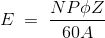• N = 60A E / PZØ
• N = E / kØ

where,

• k = PZ/60A
• N = V – Ia Ra / kØ

Therefore speed (N) of 3 types of DC motor – SERIES, SHUNT and COMPOUND can be controlled by changing the quantities on RHS of the expression. So speed can be varied by changing

1. Terminal voltage of the armature V.
2. External resistance in armature circuit Ra.
3. Flux per pole φ.

The first two cases involve change that affects armature circuit and the third one involves change in magnetic field. Therefore speed control of DC motor is classified as:

1. Armature control methods
2. Field control methods.

### Speed Control of DC Series Motor

Speed control of DC series motor can be done either by armature control or by field control.

#### Armature Control of DC Series Motor

Speed adjustment of DC series motor by armature control may be done by any one of the methods that follow,

1. Armature Resistance Control Method:

This is the most common method employed. Here the controlling resistance is connected directly in series with the supply of the motor as shown in the fig.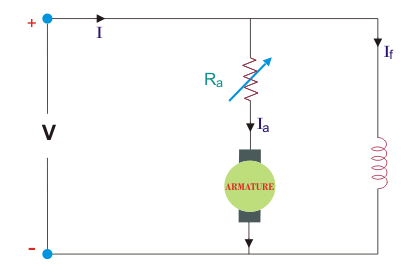The power loss in the control resistance of DC series motor can be neglected because this control method is utilized for a large portion of time for reducing the speed under light load condition.

This method of speed control is most economical for constant torque. This method of speed control is employed for DC series motor driving cranes, hoists, trains etc.

2. Shunted Armature Control

The combination of a rheostat shunting the armature and a rheostat in series with the armature is involved in this method of speed control. The voltage applied to the armature is varies by varying series rheostat R1.

The exciting current can be varied by varying the armature shunting resistance R2. This method of speed control is not economical due to considerable power losses in speed controlling resistances. Here speed control is obtained over wide range but below normal speed.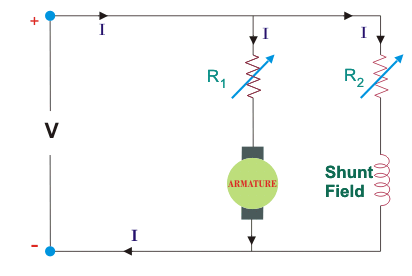3. Armature terminal voltage control

The speed control of DC series motor can be accomplished by supplying the power to the motor from a separate variable voltage supply. This method involves high cost so it rarely used.

#### Field Control of DC Series Motor

The speed of DC motor can be controlled by this method by any one of the following ways:

1. Field Diverter Method

This method uses a diverter. Here the field flux can be reduced by shunting a portion of motor current around the series field. Lesser the diverter resistance less is the field current, less flux therefore more speed. This method gives speed above normal and the method is used in electric drives in which speed should rise sharply as soon as load is decreased.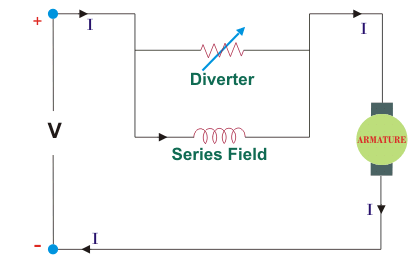2. Tapped Field Control

This is another method of increasing the speed by reducing the flux and it is done by lowering number of turns of field winding through which current flows. In this method a number of tapping from field winding are brought outside. This method is employed in electric traction.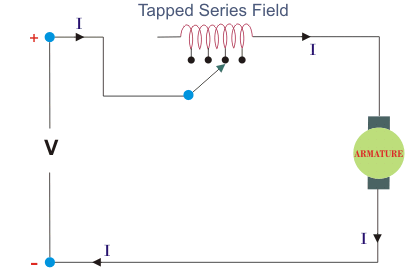### Speed Control of DC Shunt Motor

Speed of DC shunt motor is controlled by the factors stated below:

#### Field Control of DC Shunt Motor

By this method speed control is obtained by any one of the following means:

##### 1. Field Rheostat Control of DC Shunt Motor

In this method, speed variation is accomplished by means of a variable resistance inserted in series with the shunt field. An increase in controlling resistances reduces the field current with a reduction in flux and an increase in speed.

This method of speed control is independent of load on the motor. Power wasted in controlling resistance is very less as field current is a small value. This method of speed control is also used in DC compound motor.

Limitations of this Method of Speed Control

• Creeping speeds cannot be obtained.
• Top speeds only obtained at reduced torque.
• The speed is maximum at minimum value of flux, which is governed by the demagnetizing effect of armature reaction on the field.
##### 2. Field Voltage Control

This method requires a variable voltage supply for the field circuit which is separated from the main power supply to which the armature is connected. Such a variable supply can be obtained by an electronic rectifier.

#### Armature Control of DC Shunt Motor

Speed control by this method involves two ways. These are:

##### 1. Armature Resistance Control

In this method armature circuit is provided with a variable resistance. Field is directly connected across the supply so flux is not changed due to variation of series resistance. This is applied for DC shunt motor. This method is used in printing press, cranes, hoists where speeds lower than rated is used for a short period only.

##### 2. Armature Voltage Control

This method of speed control needs a variable source of voltage separated from the source supplying the field current. This method avoids disadvantages of poor speed regulation and low efficiency of armature-resistance control methods.

The basic adjustable armature voltage control method of speed d control is accomplished by means of an adjustable voltage generator is called Ward Leonard System. This method involves using a motor-generator (M-G) set. This method is best suited for steel rolling mills, paper machines, elevators, mine hoists, etc. This method is known as Ward Leonard System.

1. Very fine speed control over whole range in both directions
2. Uniform acceleration is obtained
3. Good speed regulation
4. It has regenerative braking capacity